幕墙,玻璃幕墙,建筑幕墙,幕墙公司,幕墙装饰,幕墙设计,门窗幕墙,幕墙玻璃,点式幕墙,铝幕墙,点式玻璃幕墙,幕墙规范,幕墙材料,玻璃幕墙工程技术规范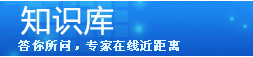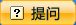知识库首页 >> 精彩词条 >> 幕墙结构计算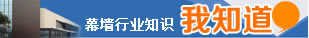### 精彩词条# 幕墙结构计算

qek1=qekt13/(t13+t23)=0.147×63/(63+63)=0.07(kPa)

qek2=qekt23/(t13+t23)=0.147×63/(63+63)=0.07(kPa)

σek1=6mqek1a2/t12=6×0.0868×0.07×12002/62=1.46(MPa)

σek2=6mqek2a2/t22=6×0.0868×0.07×12002/62=1.46(MPa){TodayHot}
4.5.1.3应力折减系数
θ1=(wk1+0.5qek1)a4/(Egt14)=(1.14+0.5×0.07)×10-3×12004/(7.2×104×64)=26.1
θ2=(wk2+0.5qek2)a4/(Egt24)=(1.14+0.5×0.07)×10-3×12004/(7.2×104×64)=26.1

4.5.1.4应力组合设计值

σ1=η1(ψwγwσwk1+ψeγeσek1)=0.896×(1.0×1.4×23.75+0.5×1.3×1.46)=30.64(MPa)

### 其他补充

 我来补充： 回答即可得分。若被选为最佳答案，您可获得更多奖励分。 马上登录 没帐号?马上注册

注册登录后回答，赢取积分！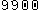4734 词条

### 热门词条榜 [全部词条]

1. 1结构胶
2. 2建筑幕墙
3. 3铝合金
4. 4密封胶
5. 5单元式幕墙
6. 6玻璃胶
7. 7钛合金板
8. 8
9. 9彩色钢板
10. 10NOBODY发布的更多词条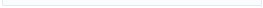相关词条